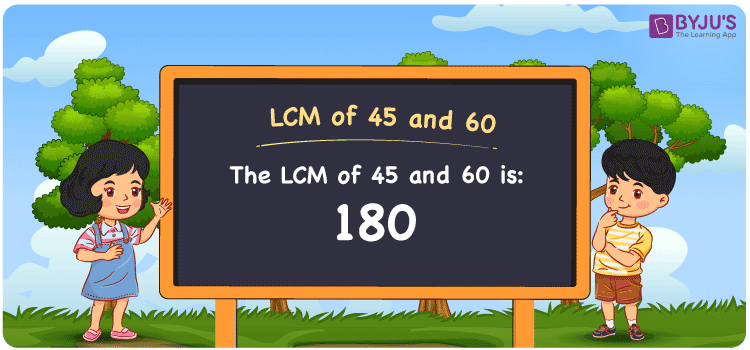Checkout JEE MAINS 2022 Question Paper Analysis : Checkout JEE MAINS 2022 Question Paper Analysis :

# LCM of 45 and 60

LCM of 45 and 60 is 180. The value divisible evenly by 45 and 60 gives the LCM value. Least common multiples of 45 and 60 can be found from the multiples which are common. (45, 90, 135, 180, 225, 270, ….) and (60, 120, 180, 240, 300, 360,….) are the multiples of 45 and 60. Students will learn how LCM of two numbers can be found using prime factorization, division and by listing the multiples using this article as a reference.

## What is LCM of 45 and 60?

The answer to this question is 180. The LCM of 45 and 60 using various methods is shown in this article for your reference. The LCM of two non-zero integers, 45 and 60, is the smallest positive integer 180 which is divisible by both 45 and 60 with no remainder.## How to Find LCM of 45 and 60?

LCM of 45 and 60 can be found using three methods:

• Prime Factorisation
• Division method
• Listing the multiples

### LCM of 45 and 60 Using Prime Factorisation Method

The prime factorisation of 45 and 60, respectively, is given by:

45 = 3 x 3 x 5 = 3² x 5

60 = 2 x 2 x 3 x 5 = 2² x 3¹ x 5¹

LCM (45, 60) = 180

### LCM of 45 and 60 Using Division Method

We’ll divide the numbers (45, 60) by their prime factors to get the LCM of 45 and 60 using the division method (preferably common). The LCM of 45 and 60 is calculated by multiplying these divisors.

 2 45 60 2 45 30 3 45 15 3 15 5 5 5 5 x 1 1

No further division can be done.

Hence, LCM (45, 60) = 180

### LCM of 45 and 60 Using Listing the Multiples

To calculate the LCM of 45 and 60 by listing out the common multiples, list the multiples as shown below

 Multiples of 45 Multiples of 60 45 60 90 120 135 180 180 240 225 – 270 –

LCM (45, 60) = 180

## Video Lesson on Applications of LCM## LCM of 45 and 60 Solved Examples

Which is the smallest number divisible by 45 and 60 exactly?

Solution:

The smallest number divisible by 45 and 60 exactly is the LCM.

Multiples of 45 = 45, 90, 135, 180, 225, ….

Multiples of 60 = 60, 120, 180, 240, 300, …..

Hence, the LCM of 45 and 60 is 180.

## Frequently Asked Questions on LCM of 45 and 60

### What is the LCM of 45 and 60?

The LCM of 45 and 60 is 180.

### What is the GCF if the LCM of 45 and 60 is 180?

LCM x GCF = 45 x 60

Given

LCM of 45 and 60 = 180

180 x GCF = 2700

GCF = 2700/180 = 15

### What is the relation between GCF and LCM of 45 and 60?

The relation between GCF and LCM of 45 and 60 is

LCM x GCF = 45 x 60

LCM x GCF = 2700